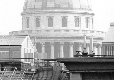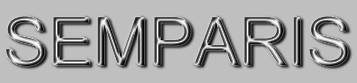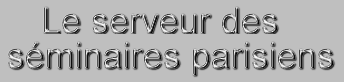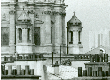The SEMPARIS seminar webserver hosts annoucements of all seminars taking place in Paris area, in all topics of physics, mathematics and computer science. It allows registered users to receive a selection of announcements by email on a daily or weekly basis, and offers the possibility to archive PDF or Powerpoint files, making it available to the scientific community.   [ More information ]

 Upcoming Seminars [Next 30 ] [ scheduler view ]

 Thursday 29 September 2022, 12:45 at LPENS, room L367 LPENS-BQ (Balades Quantiques de le LPENS) cond-mat Rosario Fazio ( ICTP (Trieste) ) Symmetry breaking and Entanglement transitions in driven-dissipative systems Abstract: Quantum systems evolving unitarily and subject to quantum measurements exhibit various types of non- equilibrium phase transitions, arising from the competition between unitary evolution and measurements. Dissipative phase transitions in steady states of time-independent Liouvillians and measurement induced phase transitions at the level of quantum trajectories are two primary examples of such transitions. Investigating a many-body spin system subject to periodic resetting measurements, we argue that many- body dissipative Floquet dynamics provides a natural framework to analyze both types of transitions. We show that a dissipative phase transition between a ferromagnetic ordered phase and a paramagnetic disordered phase emerges for long-range systems as a function of measurement probabilities. A measurement induced transition of the entanglement entropy between volume law scaling and sub-volume law scaling is also present, and is distinct from the ordering transition. The two phases correspond to an error-correcting and a quantum-Zeno regimes, respectively. The ferromagnetic phase is lost for short range interactions, while the volume law phase of the entanglement is enhanced.

 Thursday 29 September 2022, 14:00 at LPTM, 4.13b + Teams distanciel SEM-LPTM-UCP (Seminaires du LPTM , Universite de Cergy Pontoise) cond-mat Dima Kovrizhin ( LPTM CY Cergy Paris Université ) Current correlations out of equilibrium in an electron collider Abstract: Recent experiments with quantum Hall edge states allowed one to measure current correlations in an electron collider, where a pair of non-equilibrium edge states are scattered across a quantum point contact. In the fractional quantum Hall regime this setting offered a possibility to test anyon statistics of quasiparticles. Remarkably, in the integer quantum Hall regime at \nu=2 when the system is driven out of equilibrium by a finite bias voltage, the measurements showed unexpected results. In this talk I will discuss a theoretical model of these experiments, and will present an exact solution of this model which explains the experimental observations.

 Friday 30 September 2022, 10:00 at IPHT, Salle Claude Itzykson, Bât. 774 COURS (Cours) physics Bertrand Eynard ( IPhT ) Introduction to Topological Recursion Abstract: Videoconference: subscribe to the course newsletter to receive links Abstract: \\ Topological Recursion is a mathematical tool. From an initial data S, called the spectral curve, the recursion produces a sequence $\omega_{g,n}(S)$ indexed by two integers g,n. These sequences have many applications that range from string theory to random matrices, statistical physics on a random lattice, integrable systems, WKB asymptotics, CFT, ... We shall introduce Topological Recursion by examples and concrete applications, and mention some long-reach issues. \\ Plan: \\ 1) Introduction by examples of spectral curves: random matrix spectral densities (semi-circle $y=\sqrt{1-x^2}$), the Witten-Kontsevich curve ($y=\sqrt{x}$), and the Mirzakhani's curve ($y=\sin\sqrt{x}$), and their applications, in particular the volumes of the space of hyperbolic surfaces, the Mirzakhani's recursion. \\ 2) Going from examples to general Topological Recursion. Practical methods for computing Topological Recursion, in particular graphical methods, and general properties. \\ 3) Link to the geometry of surfaces: moduli space of Riemann surfaces, cohomological field theories, towards string theory. \\ 4) Topological Recursion as a powerful method to compute WKB series. Link to differential equations and integrable systems.

 Friday 30 September 2022, 14:15 at IPHT, Salle Claude Itzykson, Bât. 774 IPHT-MAT (Séminaire de matrices, cordes et géométries aléatoires) hep-th Severin Lüst ( Harvard ) Holography and the KKLT Scenario Abstract: The KKLT scenario, one of the few ideas to realize dS vacua in string theory, consists of two steps: the first involves the construction of a supersymmetric AdS vacuum with a small negative cosmological constant, and the second involves breaking supersymmetry and uplifting the energy to achieve dS. In this talk I discuss conventional holography to argue why it is not possible to complete the first step. This is obtained this by putting a bound on the central charge of the dual theory which involves branes wrapping special Lagrangian cycles in CY 4-folds.

 Monday 3 October 2022, 14:00 at IHES, Amphithéâtre Léon Motchane ( Séminaire Géométrie et groupes discrets ) MATH-IHES (TBA) math Serge Cantat ( CNRS & Université de Rennes 1 ) Elementary Properties of Groups of Polynomial Automorphisms Abstract: Let G be a finitely generated group acting faithfully by linear transformations on a finite-dimensional complex vector space. The theorems of Malcev, Selberg, or Tits provide important properties satisfied by G. To what extent do these properties continue to hold when G is acting by polynomial (instead of linear) transformations? In order to address this question, I shall describe a few results that illustrate how one can use p-adic or finite fields for problems which are initially phrased in terms of complex numbers.

 Monday 3 October 2022, 14:00 at LPENS, L369, 24 rue Lhomond ( 3 lecture mini-course. Each lecture 2 hours. In-person only ) LPTENS-HE (Séminaire commun LPTENS-LPTHE) hep-th Marcos Mariño ( University of Geneva ) An introduction to resurgence in quantum theory Abstract: In quantum theory, we often calculate observables approximately by using perturbative series in a small parameter. These series are typically factorially divergent, so we need to make sense of them. The theory of resurgence gives a general framework to do this, and upgrades perturbative series to so- called trans-series, which include exponentially small corrections and make it possible to incorporate non-perturbative physics in a systematic way. Many non-perturbative techniques in quantum theory (like instanton calculus and renormalon physics) are in fact particular examples of this general framework. In these lectures I will give an introduction to resurgence with a focus on applications to quantum theory. After introducing some elementary resurgent technology, I will discuss resurgence in one-dimensional quantum mechanics, and then proceed to discuss what is known about the resurgent structure of quantum field theory.

 Monday 3 October 2022, 14:00 at LPTM, 4.13b + Teams distanciel SEM-LPTM-UCP (Seminaires du LPTM , Universite de Cergy Pontoise) math-ph Q Charles Su ( Department of Physics, Illinois State University , Normal IL 61790 USA ) Classical, QFT, and QED solutions of the Dirac equation Abstract: The computational quantum field theory developed by Dr. Sus research team provides an effective means for studying the vacuum breakdown by ultra-strong fields and the generation of electron-positron pairs with spatial and temporal resolution. With the help of this computational tool, we were able to comment on the Klein paradox. More recently, we have focused on how to effectively lower the field threshold to trigger pair creation by designing external field configurations with optimal variations in space or in time. We have extended our model to investigate the origin of the Coulomb force where electron-proton interactions are facilitated not through an external background field but through the exchange of mediating photons. The present talk discusses some results obtained by solving the Dirac equation from the perspectives of classical mechanics, quantum field theory, and quantum electrodynamics.

 Monday 3 October 2022, 16:00 at IHES, Amphithéâtre Léon Motchane ( Séminaire Géométrie et groupes discrets ) MATH-IHES (TBA) math Cagri Sert ( Universität Zürich ) Stationary Measures for SL(2,R)-actions on Homogeneous Bundles over Flag Varieties Abstract: Let $X_{k,d}$ denote the space of rank-k lattices in $R^d$. Topological and statistical properties of the dynamics of discrete subgroups of G=SL(d,R) on $X_{d,d}$ were described in the seminal works of Benoist-Quint. A key step/result in this study is the classification of stationary measures on $X_{d,d}$. Later, Sargent-Shapira initiated the study of dynamics on the spaces $X_{k,d}. When$k \ne d$, the space$X_{k,d}$is of a different nature and a clear description of dynamics on these spaces is far from being established. Given a probability measure$\mu$which is Zariski-dense in a copy of SL(2,R) in G, we give a classification of$\mu$-stationary measures on$X_{k,d}$and prove corresponding equidistribution results. In contrast to the results of Benoist-Quint, the type of stationary measures that$\mu$admits depends strongly on the position of SL(2,R) relative to parabolic subgroups of G. I will start by reviewing preceding major works and ideas. The talk will be accessible to a broad audience. Joint work with Alexander Gorodnik and Jialun Li.  Tuesday 4 October 2022, 14:00 at LPTMS, Salle des séminaires du FAST et du LPTMS, bâtiment Pascal n°530 ( Hybrid: onsite seminar + zoom. For zoom info, please write to valentina.ros@universite-paris-saclay.fr or check LPTMS website ) LPTMS (Séminaire du Laboratoire de Physique Théorique et Modèles Statistiques (Orsay)) cond-mat.stat-mech Emilio Trevisani ( IPhT ) Random field models and Parisi-Sourlas supersymmetry Abstract: Quenched disorder is very important but notoriously hard. In 1979, Parisi and Sourlas proposed an interesting and powerful conjecture about the infrared fixed points with random field (RF) type of disorder: such fixed points should possess an unusual supersymmetry, by which they reduce in two less spatial dimensions to usual non- supersymmetric non-disordered fixed points. The conjecture is known to hold for the RF phi^3 model but not for RF phi^4 model in d < 5 dimensions, however there is no consensus on why this happens. We argue that: 1) dimensional reduction works for any Parisi-Sourlas SUSY fixed point; 2) the SUSY fixed point is not always reached because of new relevant SUSY-breaking interactions. We attack the point 1) using axiomatic CFT techniques while we study the point 2) using the perturbative renormalization group in a judiciously chosen field basis, allowing systematic exploration of the space of interactions. Our computations agree with the numerical results for both cubic and quartic potential.  Wednesday 5 October 2022, 13:30 at DPT-PHYS-ENS, ConfIV (E244) - 24 rue Lhomond 75005 PARIS COLLOQUIUM-ENS (Colloquium of the Physics Department of ENS) physics Timo Weigand ( Hamburg ) TBA Abstract: TBA  Thursday 6 October 2022, 12:45 at LPENS, L367 LPENS-BQ (Balades Quantiques de le LPENS) cond-mat Chaitanya Murthy Tba Abstract: tba  Thursday 6 October 2022, 14:00 at LPTM, Amphi E1 St Martin SOUTEN-TH (Soutenance de thèse) math-ph Mohammed Alkhateeb ( LPTM CY Cergy Paris Université ) Klein Tunneling for Fermions and Bosons in First and Second Quantization: Space and Time Resolved Approaches  Friday 7 October 2022, 09:01 at IPHT, Salle Claude Itzykson, Bât. 774 COURS (Cours) physics Bertrand Eynard ( IPhT ) Introduction to Topological Recursion Abstract: Videoconference: subscribe to the course newsletter to receive links Abstract: \\ Topological Recursion is a mathematical tool. From an initial data S, called the spectral curve, the recursion produces a sequence$\omega_{g,n}(S)$indexed by two integers g,n. These sequences have many applications that range from string theory to random matrices, statistical physics on a random lattice, integrable systems, WKB asymptotics, CFT, ... We shall introduce Topological Recursion by examples and concrete applications, and mention some long-reach issues. \\ Plan: \\ 1) Introduction by examples of spectral curves: random matrix spectral densities (semi-circle$y=\sqrt{1-x^2}$), the Witten-Kontsevich curve ($y=\sqrt{x}$), and the Mirzakhani's curve ($y=\sin\sqrt{x}$), and their applications, in particular the volumes of the space of hyperbolic surfaces, the Mirzakhani's recursion. \\ 2) Going from examples to general Topological Recursion. Practical methods for computing Topological Recursion, in particular graphical methods, and general properties. \\ 3) Link to the geometry of surfaces: moduli space of Riemann surfaces, cohomological field theories, towards string theory. \\ 4) Topological Recursion as a powerful method to compute WKB series. Link to differential equations and integrable systems.  Monday 10 October 2022, 11:00 at IPHT, Salle Claude Itzykson, Bât. 774 IPHT-PHM (Séminaire de physique mathématique) math-ph Kirone Mallick ( IPhT ) An exact solution of the macroscopic fluctuation theory Abstract: Interacting diffusive particle systems are paradigms for non-equilibrium statistical physics. Their macroscopic behaviour follows a variational principle, proposed by G. Jona-Lasinio and his collaborators, known as the Macroscopic Fluctuation Theory (MFT), in which physics out from equilibrium is determined at a coarse-grained scale by two coupled non-linear hydrodynamic equations. In this talk, we shall show that the MFT equations for the exclusion process are classically integrable, i.e. they can be integrated by inverse scattering, a method originally used to study solitons in the KdV or the NLS equations. Our exact solution will allow us to understand how large deviations are generated by atypical fluctuations, far from equilibrium. [The talk will also be streamed online, please ask the organizers for the link.]  Tuesday 11 October 2022, 11:00 at LPTMS, Salle des séminaires du FAST et du LPTMS, bâtiment Pascal n°530 ( Hybrid: onsite seminar + zoom. For zoom info, please write to valentina.ros@universite-paris-saclay.fr or check LPTMS website. ) LPTMS (Séminaire du Laboratoire de Physique Théorique et Modèles Statistiques (Orsay)) cond-mat.stat-mech Guillaume Barraquand ( Ècole Normale Supérieure Paris ) Stationary measures for the KPZ equation Abstract: A solution h(x,t) of the KPZ equation in one dimension typically grows linearly in time with t^{1/3} fluctuations. The height gradient, however, or the differences of height between any two points, will reach a stationary state. In that sense, it has been known for a long time that the Brownian motion is a stationary measure for the KPZ equation on the full-line. For domains with boundaries such as [0,L] or R_+, stationary measures were characterized only recently using a combination of works by several groups, and can be described using Brownian motions reweighted by exponential functionals. The talk is based on joint works with Pierre Le Doussal and Ivan Corwin.  Wednesday 12 October 2022, 14:00 at LPENS, L363, 24 rue Lhomond ( 3 lecture mini-course. Each lecture 2 hours. In-person only ) LPTENS-HE (Séminaire commun LPTENS-LPTHE) hep-th Marcos Mariño ( University of Geneva ) An introduction to resurgence in quantum theory Abstract: In quantum theory, we often calculate observables approximately by using perturbative series in a small parameter. These series are typically factorially divergent, so we need to make sense of them. The theory of resurgence gives a general framework to do this, and upgrades perturbative series to so- called trans-series, which include exponentially small corrections and make it possible to incorporate non-perturbative physics in a systematic way. Many non-perturbative techniques in quantum theory (like instanton calculus and renormalon physics) are in fact particular examples of this general framework. In these lectures I will give an introduction to resurgence with a focus on applications to quantum theory. After introducing some elementary resurgent technology, I will discuss resurgence in one-dimensional quantum mechanics, and then proceed to discuss what is known about the resurgent structure of quantum field theory.  Wednesday 12 October 2022, 14:15 at IPHT, Salle Claude Itzykson, Bât. 774 IPHT-MAT (Séminaire de matrices, cordes et géométries aléatoires) hep-th Gustavo J. Turiaci ( IAS ) TBA Abstract: TBA  Thursday 13 October 2022, 09:00 at LPTENS, Salle Jaurès, ENS Paris ( https://sites.google.com/view/parisiday-ens/home register at http://www.phys.ens.fr/spip.php?article5622 ) WORK-CONF (Workshop or Conference) hep-th Two Days With Giorgio Parisi In Paris TBA  Thursday 13 October 2022, 11:00 at LPTHE, bibliothèque du LPTHE, tour 13-14, 4eme étage SEM-DARBOUX (Séminaire Darboux - physique théorique et mathématiques) hep-th|math.AG Susanna Zimmermann ( Laboratoire de Mathématiques d'Orsay, Université Paris-Saclay ) Birational maps of the plane Abstract: Birational symmetries are among the oldest in literature, starting with the involution at the circle by Apollonius. A birational map is simply a map whose coordinates are quotients of polynomials and that have an inverse map of the same type. The group of birational maps of the plane is very large and has enjoyed attention on and off for about 150 years. In this talk I will give an overview of some recent advances.  Friday 14 October 2022, 09:00 at LPTENS, Salle Jaurès, ENS Paris ( https://sites.google.com/view/parisiday-ens/home register at http://www.phys.ens.fr/spip.php?article5622 ) WORK-CONF (Workshop or Conference) hep-th Two Days With Giorgio Parisi In Paris TBA  Friday 14 October 2022, 10:00 at IPHT, Salle Claude Itzykson, Bât. 774 COURS (Cours) physics Bertrand Eynard ( IPhT ) Introduction to Topological Recursion Abstract: Videoconference: subscribe to the course newsletter to receive links Abstract: \\ Topological Recursion is a mathematical tool. From an initial data S, called the spectral curve, the recursion produces a sequence$\omega_{g,n}(S)$indexed by two integers g,n. These sequences have many applications that range from string theory to random matrices, statistical physics on a random lattice, integrable systems, WKB asymptotics, CFT, ... We shall introduce Topological Recursion by examples and concrete applications, and mention some long-reach issues. \\ Plan: \\ 1) Introduction by examples of spectral curves: random matrix spectral densities (semi-circle$y=\sqrt{1-x^2}$), the Witten-Kontsevich curve ($y=\sqrt{x}$), and the Mirzakhani's curve ($y=\sin\sqrt{x}$), and their applications, in particular the volumes of the space of hyperbolic surfaces, the Mirzakhani's recursion. \\ 2) Going from examples to general Topological Recursion. Practical methods for computing Topological Recursion, in particular graphical methods, and general properties. \\ 3) Link to the geometry of surfaces: moduli space of Riemann surfaces, cohomological field theories, towards string theory. \\ 4) Topological Recursion as a powerful method to compute WKB series. Link to differential equations and integrable systems.  Tuesday 18 October 2022, 11:00 at LPTMS, Salle des séminaires du FAST et du LPTMS, bâtiment Pascal n°530 ( Hybrid: onsite seminar + zoom. For zoom info, please write to valentina.ros@universite-paris-saclay.fr or check LPTMS website ) LPTMS (Séminaire du Laboratoire de Physique Théorique et Modèles Statistiques (Orsay)) cond-mat.stat-mech David Carpentier ( Ecole Normale Supérieure de Lyon ) TBA  Wednesday 19 October 2022, 11:00 at LKB, Jussieu - Amphi Charpak - Paris 5e SEM-LKB (Séminaire du Laboratoire Kastler Brossel) quant-ph Barbara Terhal ( QuTech & EEMCS Dept. Delft University of Technology ) Quantum Error Correction: Dream or Nightmare Abstract: I will discuss the ideas behind quantum error correction. I will give an overview of some recent research on quantum error correction with superconducting qubits. In particular I will discuss the problem of qubit leakage as well as the alternative of bosonic error correction.  Wednesday 19 October 2022, 13:30 at DPT-PHYS-ENS, ConfIV (E244) - 24 rue Lhomond 75005 PARIS COLLOQUIUM-ENS (Colloquium of the Physics Department of ENS) physics Basile Gallet ( CEA saclay) ) TBA Abstract: TBA  Thursday 20 October 2022, 10:00 at LPTHE, Jussieu, Conference room 44.45.106 (access via tower 44) WORK-CONF (Workshop or Conference) math-ph Various Speakers ( Various institutions ) Workshop: Topological Quantum Phases Of Matter Beyond Two Dimensions  Thursday 20 October 2022, 11:00 at IHES, Amphithéâtre Léon Motchane MATH-IHES (TBA) math Dmitry Kubrak ( IHES ) Hodge-proper Stacks and Totaro's Conjecture, part 1 Abstract: I will talk about a series of works with Artem Prikhodko where we develop a version of p-adic Hodge theory in the setting of Artin stacks. One of the main motivations for our project was a conjecture by Totaro: namely, based on his concrete computations, he suggested that the dimension of mod p de Rham cohomology of the classifying stack BG for G reductive might always be bounded from below by the dimension of the Fp-singular cohomology on the classifying space BG(C) of the Lie group G(C) of complex points of G. For smooth and proper schemes such an inequality is a consequence of integral p-adic Hodge theory in the form proved by Bhatt-Morrow-Scholze; however, their results can not be applied here directly since BG is not proper. To prove Totaro's conjecture, using the theory of prismatic cohomology, we develop integral p-adic Hodge theory in a more general setting of Hodge-proper stacks: these are stacks that only look proper from the point of view of its Hodge cohomology. However, one problem then still remains: namely, the étale comparison we get is with the étale cohomology of the Raynaud generic fiber, which a priori agrees with the algebraic generic fiber (and then complex points) only in the smooth proper setting. Nevertheless, we prove that the two étale cohomology theories still agree at least for quotient stacks [X/G] with X smooth and proper and G reductive. This then implies Totaro's conjecture by plugging X=pt. In further work we also show that after inverting p the two étale cohomology agree for any Hodge-proper stack, which sets up rational p-adic Hodge theory (with the crystalline and de Rham comparisons, and Hodge-Tate decomposition) in this setting. If time permits, I will also tell about some explicit computations of cohomology of reductive groups in characteristic p that one can perform using the above comparison in the case of BG (this is a series of joint works with Federico Scavia and Anlong Chua).  Friday 21 October 2022, 10:00 at IPHT, Salle Claude Itzykson, Bât. 774 COURS (Cours) physics Bertrand Eynard ( IPhT ) Introduction to Topological Recursion Abstract: Videoconference: subscribe to the course newsletter to receive links Abstract: \\ Topological Recursion is a mathematical tool. From an initial data S, called the spectral curve, the recursion produces a sequence$\omega_{g,n}(S)$indexed by two integers g,n. These sequences have many applications that range from string theory to random matrices, statistical physics on a random lattice, integrable systems, WKB asymptotics, CFT, ... We shall introduce Topological Recursion by examples and concrete applications, and mention some long-reach issues. \\ Plan: \\ 1) Introduction by examples of spectral curves: random matrix spectral densities (semi-circle$y=\sqrt{1-x^2}$), the Witten-Kontsevich curve ($y=\sqrt{x}$), and the Mirzakhani's curve ($y=\sin\sqrt{x}\$), and their applications, in particular the volumes of the space of hyperbolic surfaces, the Mirzakhani's recursion. \\ 2) Going from examples to general Topological Recursion. Practical methods for computing Topological Recursion, in particular graphical methods, and general properties. \\ 3) Link to the geometry of surfaces: moduli space of Riemann surfaces, cohomological field theories, towards string theory. \\ 4) Topological Recursion as a powerful method to compute WKB series. Link to differential equations and integrable systems.

 Friday 21 October 2022, 10:00 at LPTHE, Jussieu, Conference room 44.45.106 (access via tower 44) WORK-CONF (Workshop or Conference) math-ph Various Speakers ( Various institutions ) Workshop: Topological Quantum Phases Of Matter Beyond Two Dimensions

 Thursday 27 October 2022, 11:00 at IHES, Amphithéâtre Léon Motchane MATH-IHES (TBA) math Dmitry Kubrak ( IHES ) Hodge-proper Stacks and Totaro's Conjecture, part 2 Abstract: I will talk about a series of works with Artem Prikhodko where we develop a version of p-adic Hodge theory in the setting of Artin stacks. One of the main motivations for our project was a conjecture by Totaro: namely, based on his concrete computations, he suggested that the dimension of mod p de Rham cohomology of the classifying stack BG for G reductive might always be bounded from below by the dimension of the Fp-singular cohomology on the classifying space BG(C) of the Lie group G(C) of complex points of G. For smooth and proper schemes such an inequality is a consequence of integral p-adic Hodge theory in the form proved by Bhatt-Morrow-Scholze; however, their results can not be applied here directly since BG is not proper. To prove Totaro's conjecture, using the theory of prismatic cohomology, we develop integral p-adic Hodge theory in a more general setting of Hodge-proper stacks: these are stacks that only look proper from the point of view of its Hodge cohomology. However, one problem then still remains: namely, the étale comparison we get is with the étale cohomology of the Raynaud generic fiber, which a priori agrees with the algebraic generic fiber (and then complex points) only in the smooth proper setting. Nevertheless, we prove that the two étale cohomology theories still agree at least for quotient stacks [X/G] with X smooth and proper and G reductive. This then implies Totaro's conjecture by plugging X=pt. In further work we also show that after inverting p the two étale cohomology agree for any Hodge-proper stack, which sets up rational p-adic Hodge theory (with the crystalline and de Rham comparisons, and Hodge-Tate decomposition) in this setting. If time permits, I will also tell about some explicit computations of cohomology of reductive groups in characteristic p that one can perform using the above comparison in the case of BG (this is a series of joint works with Federico Scavia and Anlong Chua).

 Thursday 27 October 2022, 14:00 at LPTHE, INSP Jussieu towers 22-23 room 3-17 TQM (Theory of quantum matter) cond-mat Adam Rançon ( PhLAM Lille ) TBA Abstract: TBA

 seminars All Next Week This Week Today Tomorrow Upcoming Within a Week from series All ACFTA APC APC-COLLOQUIUM APC-TH BH-TOP BI-COSMO-IHP BI-SEM-IHP BIOPHY-ENS-ESPCI BISEMINAIRE-MP COLLOQUIUM-ENS CONDMAT-ENS CONDMAT-THEO COSMO-P6 COURS COURS-FED COURS-IPHT COURS@IAP CPHT - PHDSEM CPHT PHYS MATH CPHT- BS CPHT-JOUR CPHT-LLR CPMC DISQUANT ESPCI-COLLOQUE ESPCI/PCT FCMP FORUM-ENS FOUNDPHYS GDT-MODSTO GQ GR-COSMO IAP-SEM IDRIS-SEM IHP-ALG IHP-NONEQ IHPSTRMATH IJCLAB-COSM IMJ-AA IMJ-AUT IMJ-CHE IMJ-EAA IMJ-REP IMP-MATH-PHYS INST-ETE IPHT-DAP IPHT-GEN IPHT-HEP IPHT-MAT IPHT-PHM IPHT-SEM IPHT-STA IPN-X IPNO-DR JOUR-CLUB LP(N/T)HE LPENS-ACE LPENS-BQ LPENS-MDQ LPNHE LPS-MAGN LPS-MAT-MOL LPS-VULG LPS/ENS LPT-GEN LPT-LPTMS LPT-MAG LPT-PHYSMATH LPT-PTH LPTENS-HE LPTHE-DOC LPTHE-PPH LPTMS LPT_STAT MAG-SUPRA MAT-COND-GEN MATH-IHES MECA-STAT MOTFEYN MSC NUC-THEO PART-PHYS PHEN-PART PHYS-ESPCI PLATEAU PMMH PT-IHES P^3 RENC-THEO RENORMALISATION S-LPTENS SAMM SCOPI SEM-BESSON SEM-CPHT SEM-CSNSM SEM-DARBOUX SEM-EXCEP SEM-FED SEM-GRECO SEM-GRECO-IAP SEM-IBPC SEM-ILP SEM-INFOR SEM-INSP SEM-LAL SEM-LKB SEM-LLR SEM-LPT SEM-LPTENS SEM-LPTHE SEM-LPTM-UCP SEM-LPTMC SEM-LPTMS SEM-LUTH SEM-PHYS-ENS SEM-PMMH SEM-POINCA SEM-UPR5 SOUTEN-HDR SOUTEN-TH SPEC-LARSIM SPEC-SEM STR-LPT-ENS-HE STR-LPTHE STRINT TH-JEUX TH-MAT-COND TQM TRANSPORT TRI-SEMINAIRE WG-EXPTH-LPN/THE WORK-CONF at institute All APC CDF CITEU CPHT CSNSM CURIE DPT-PHYS-ENS ENPC ESPCI ESPCI/UPR5 GRETIA IAP IBPC IDRIS IHES IHP IJCLAB IM-JUSSIEU-PRG IMPMC INSP IPHT IPN LAL LARSIM LKB LLR LMPT LPA LPENS LPMA LPNHE LPNHE-GR-TH LPP LPS-ORSAY LPS/ENS LPT LPTENS LPTHE LPTM LPTMC LPTMS LUTH MSC OBSPARIS PCT/ESPCI PMMH SAMM SPEC UPMC in subject All CoRR -- Computing Research Repository CoRR.AI -- Artificial Intelligence CoRR.AR -- Architecture CoRR.CC -- Computational Complexity CoRR.CE -- Computational Engineering CoRR.CG -- Computational Geometry CoRR.CL -- Computation and Language CoRR.CR -- Cryptography and Security CoRR.CV -- Computer Vision and Pattern Recognition CoRR.CY -- Computers and Society CoRR.DB -- Databases CoRR.DC -- Distributed, Parallel, and Cluster Computing CoRR.DL -- Digital Libraries CoRR.DM -- Discrete Mathematics CoRR.DS -- Data Structures and Algorithms CoRR.GL -- General Literature CoRR.GR -- Graphics CoRR.GT -- Computer Science and Game Theory CoRR.HC -- Human-Computer Interaction CoRR.IR -- Information Retrieva CoRR.IT -- Information Theory CoRR.LG -- Learning CoRR.LO -- Logic in Computer Science CoRR.MA -- Multiagent Systems CoRR.MM -- Multimedia; CoRR.MS -- Mathematical Software CoRR.NA -- Numerical Analysis CoRR.NE -- Neural and Evolutionary Computing CoRR.NI -- Networking and Internet Architecture CoRR.OH -- Other CoRR.OS -- Operating Systems CoRR.PF -- Performance CoRR.PL -- Programming Languages CoRR.RO -- Robotics CoRR.SC -- Symbolic Computation CoRR.SD -- Sound CoRR.SE -- Software Engineering astro-ph -- Astrophysics cond-mat -- Condensed Matter cond-mat.dis-nn -- Disordered Sys. and Neural Networks cond-mat.mes-hall -- Mesoscopic Sys. and Q.Hall Effect cond-mat.mtrl-sci -- Materials Science cond-mat.other -- Other cond-mat.soft -- Soft Condensed Matter cond-mat.stat-mech -- Statistical Mechanics cond-mat.str-el -- Strongly Correlated Electrons cond-mat.supr-con -- Superconductivity gr-qc -- General Relativity and Quantum Cosmology hep-ex -- High Energy Physics - Experiment hep-lat -- High Energy Physics - Lattice hep-ph -- High Energy Physics - Phenomenology hep-th -- High Energy Physics - Theory math -- Mathematics math-ph -- Mathematical Physics math.AC -- Commutative Algebra math.AG -- Algebraic Geometry math.AP -- Analysis of PDEs math.AT -- Algebraic Topology math.CA -- Classical Analysis and ODEs math.CO -- Combinatorics math.CT -- Category Theory math.CV -- Complex Variables math.DG -- Differential Geometry math.DS -- Dynamical Systems math.FA -- Functional Analysis math.GM -- General Mathematics math.GN -- General Topology math.GR -- Group Theory math.GT -- Geometric Topology math.HO -- History and Overview math.KT -- K-Theory and Homology math.LO -- Logic math.MG -- Metric Geometry math.MP -- Mathematical Physics math.NA -- Numerical Analysis math.NT -- Number Theory math.OA -- Operator Algebras math.OC -- Optimization and Control math.PR -- Probability math.QA -- Quantum Algebra math.RA -- Rings and Algebras math.RT -- Representation Theory math.SG -- Symplectic Geometry math.SP -- Spectral Theory math.ST -- Statistics nlin -- Nonlinear Sciences nlin.AO -- Adaptation and Self-Organizing Systems nlin.CD -- Cellular Automata and Lattice Gases nlin.CG -- Chaotic Dynamics nlin.PS -- Exactly Solvable and Integrable Systems nlin.SI -- Pattern Formation and Solitons nucl-ex -- Nuclear Experiment nucl-th -- Nuclear Theory physics -- Physics physics.acc-ph -- Accelerator Physics physics.ao-ph -- Atmospheric and Oceanic Physics physics.atm-clus -- Atomic and Molecular Clusters physics.atom-ph -- Atomic Physics physics.bio-ph -- Biological Physics physics.chem-ph -- Chemical Physics physics.class-ph -- Classical Physics physics.comp-ph -- Computational Physics physics.data-an -- Data Analysis physics.ed-ph -- Physics Education physics.flu-dyn -- Fluid Dynamics physics.gen-ph -- General Physics physics.geo-ph -- Geophysics physics.hist-ph -- History of Physics physics.ins-det -- Instrumentation and Detectors physics.med-ph -- Medical Physics physics.optics -- Optics physics.plasm-ph -- Plasma Physics physics.pop-ph -- Popular Physics physics.soc-ph -- Physics and Society physics.space-ph -- Space Physics q-bio -- Quantitative Biology qbio.BM -- Biomolecules qbio.CB -- Cell Behavior qbio.GN -- Genomics qbio.MN -- Molecular Networks qbio.NC -- Neurons and Cognition qbio.OT -- Other qbio.PE -- Populations and Evolution qbio.QM -- Quantitative Methods qbio.SC -- Subcellular Processes; Tissues and Organs qbio.TO -- Tissues and Organs quant-ph -- Quantum Physics with field Speaker Title Abstract Subject matching

[ Postscript Poster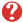| PDF Poster| RSS Thread | ICal Format]

You are invited to subscribe to SEMPARIS mailing lists in order to receive selected announcements by email.

 [ Annonces ]    [ Abonnements ]    [ Archive ]    [ Aide ]    [ JavaScript requis ] [ English version ]